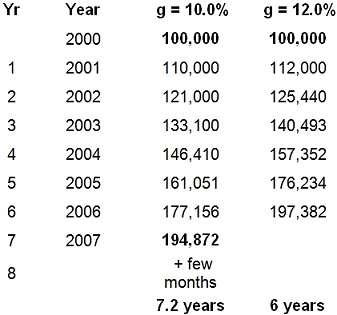BRIANWOOLF.COM

# Marketing Numbers 101

By Brian Woolf
February 1, 2015Two useful numbers for marketers and how to calculate them...

The primary purpose of marketing is to identify and meet customers needs. The outcome is, ideally, an increase in sales and profits. Marketing dreams and financial reality need, therefore, to communicate. A smart marketer is able to converse with the CEO and CFO in their language, numbers. As growth is seldom far from their minds, here are two growth measurements that may help. They are worthy additions to your Marketers Toolbox.

Rule of 72
Two common growth questions are:

• If we were able to grow sales at, say, 10% a year, how long would it take for sales to double?
• If we wanted to double sales in, say, 6 years, how much would we need to grow each year?

In the time others take to open a calculator, you can use the Rule of 72 to have the (approximate) answer to either question. Heres how:

1) When you have the growth rate and you want to know the number of years needed to double sales ...
Divide 72 by the growth rate, eg, if you expect the annual growth to be 10% per year, the answer is (approximately) 7.2 years. [Calculation: 72 / 10 = 7.2 years]

2) When you have the number of years and you want to know the growth rate needed to double sales ...
Divide 72 by the number of years, eg, if you want to know what annual % growth rate youll need to double sales in 6 years, the answer is (approximately) 12% [Calculation: 72 / 6 = 12%]

Note: see Appendix to see annual calculations*.

Average (Annual) Compound Growth Rate (acgr)
Lets assume your company has just bought another and you have been given responsibility for one of its divisions. Your CEO wants to know its most recent 4-year trajectory (ie, its average annual compound growth rate of sales) to provide a base to improve on in the next few years.

Calculating the past 4 years acgr is done with a simple formula that you enter into one cell of an Excel spreadsheet. First, gather a few pieces of data:

• The base year and the latest year (eg, 2010 and 2014)
• The number of years of growth under review (in this case 2014-2010 = 4)
• Sales in the base year and latest year (eg, \$100,000 and \$144,000)

The formula is:
=(((Latest Sales /Base Sales) ^ (1/ # Years)) -1)

Inserting the five numbers you gathered, the formula would be:
=(((144,000/100,000)^(1/4))-1)

Once entered, the acgr would immediately appear as 9.5%.

What this means is that, on average, sales grew 9.5% per year between the base year of 2010, and 2014, four years later. It does not mean than sales grew 9.5% every year; one year may have grown 16%, another 3%. It means that the average annual growth rate from the base year to the latest year was 9.5%. [Note: when selecting your formula cell, it helps to express results as percentages (%).]

The equation looks formidable, but actually its simple to set up and use. Test the above calculation yourself. Youll find it useful. For example, you can take any 5- or 10-year period and calculate, say, the acgr of your stores, sales, profits, and share price. The first three numbers usually explains the fourth.

Remember, one hard number pricks many a balloon of opinion.

* Appendix. Annual Numbers Illustrating the Rule of 72 example: